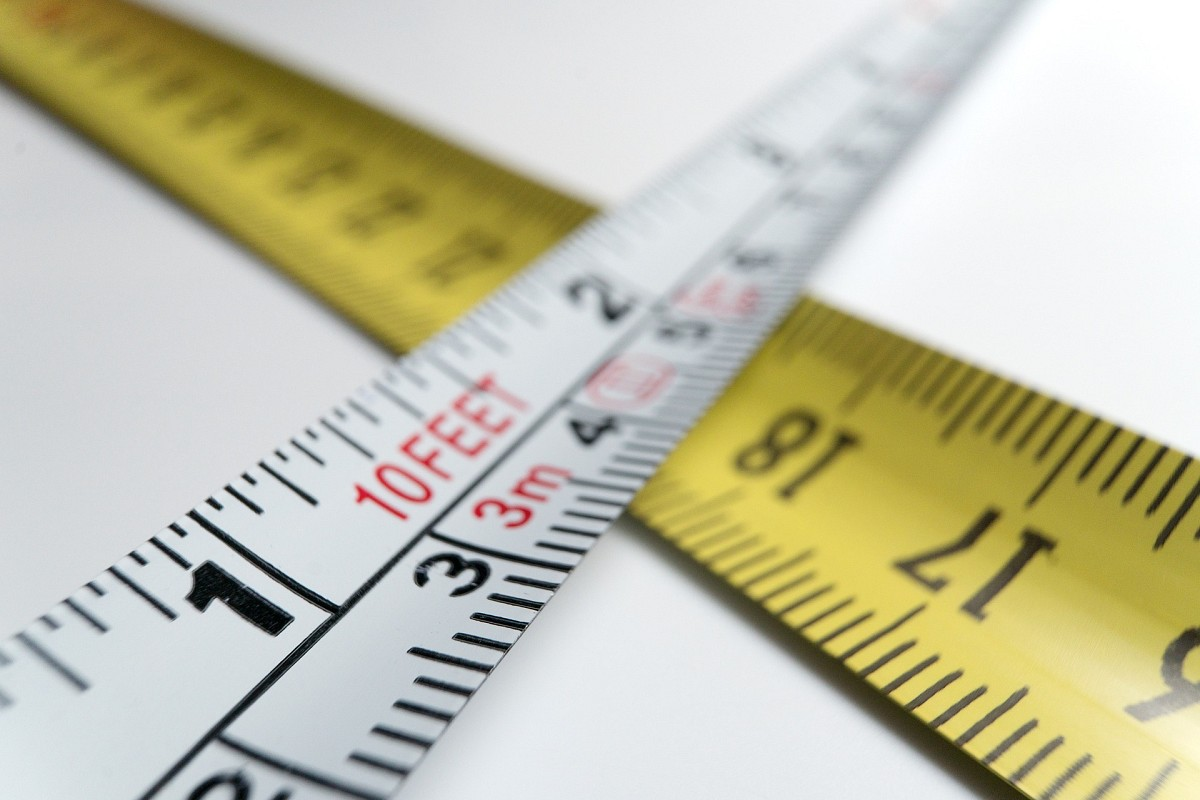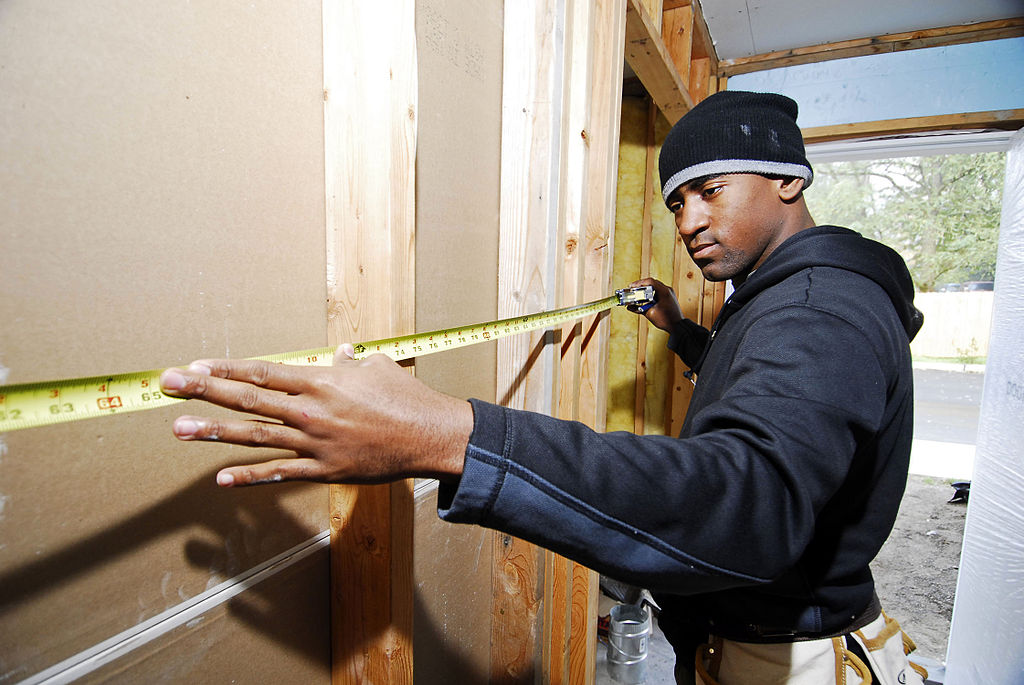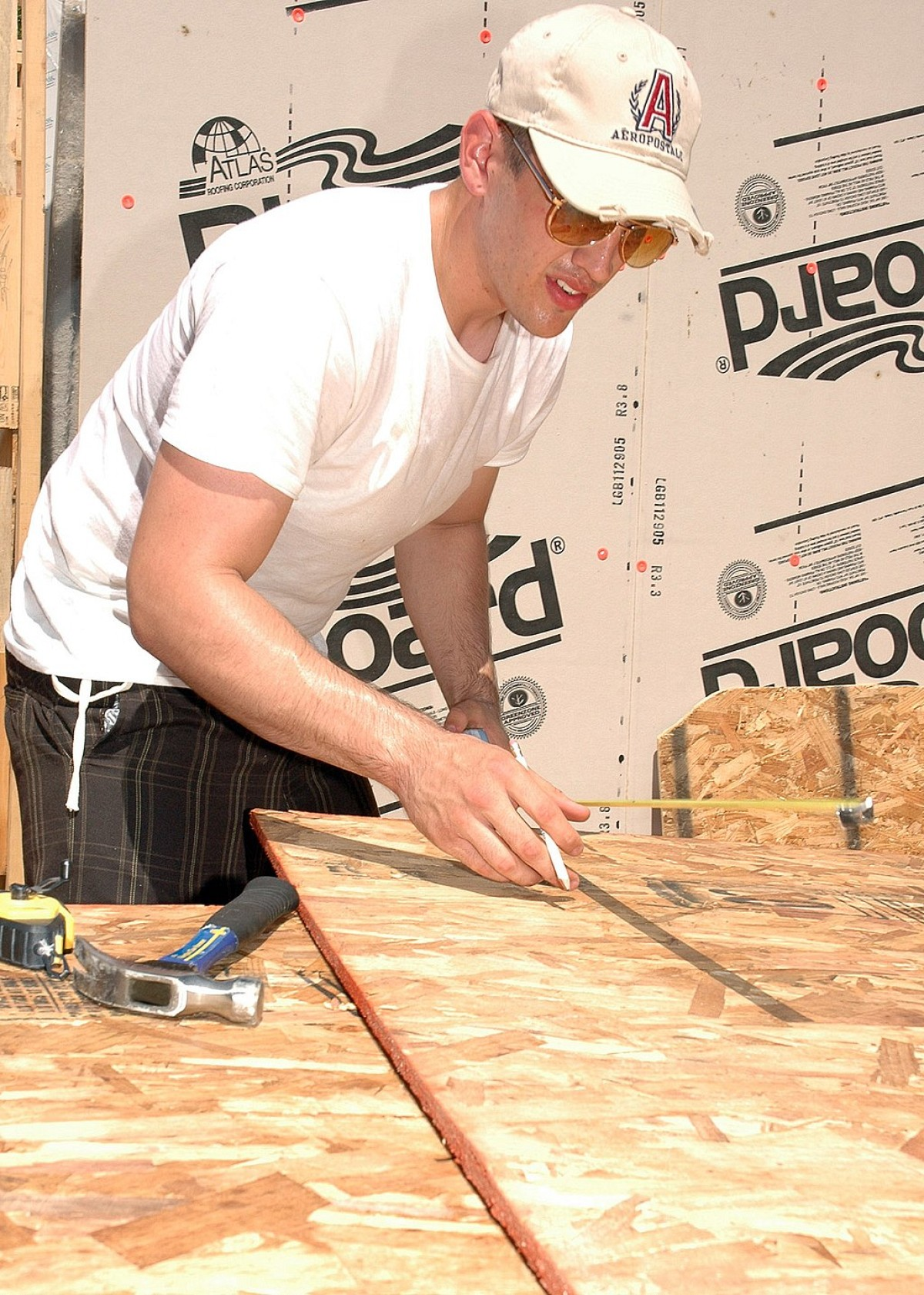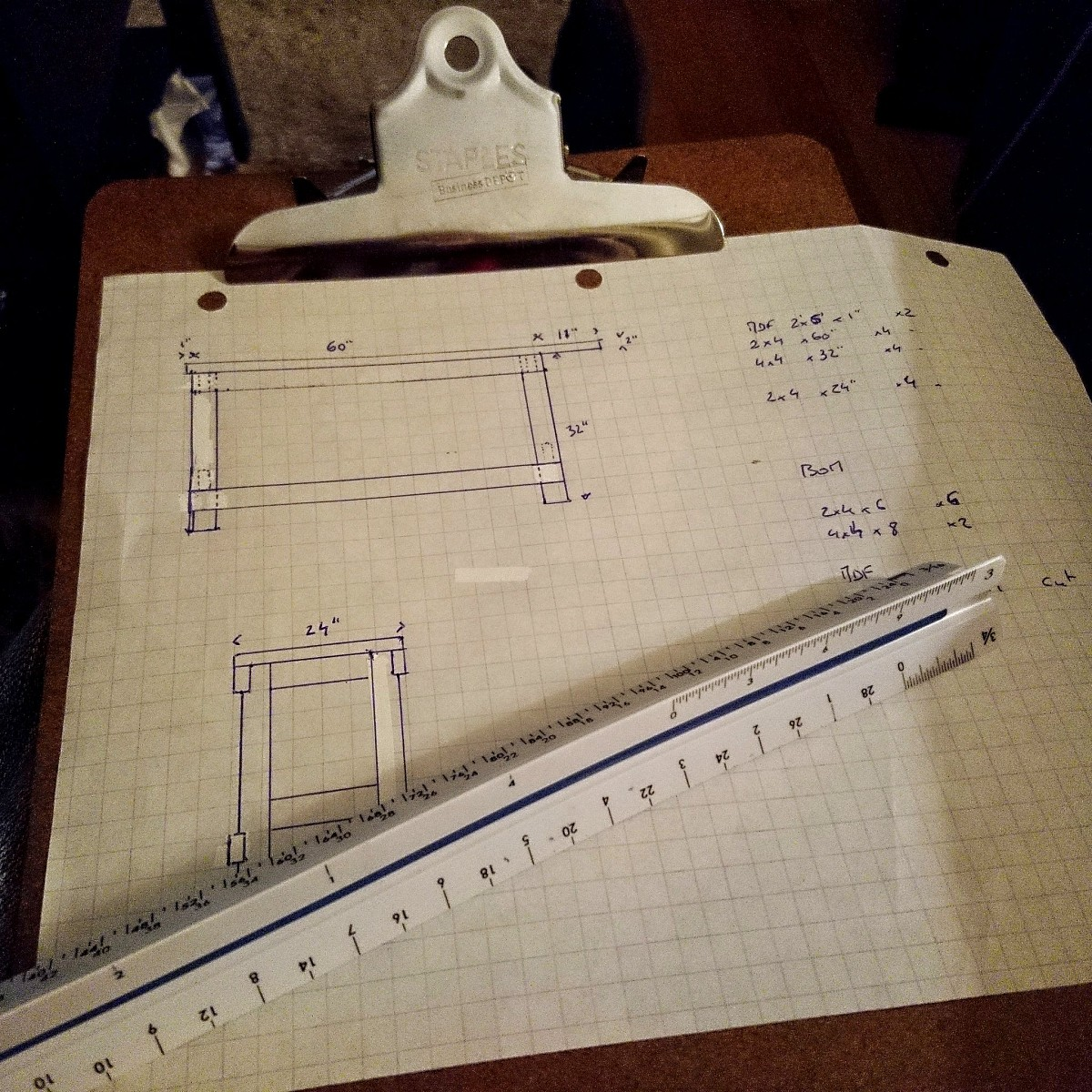# Calculate Square Footage For Home Improvement ProjectsWhat’s the most valuable home improvement skill? Learning to calculate square footage ranks right up there. If you’re not looking to rely on a remodeling contractor to size up & purchase materials on your behalf, you’ll need to master these measurements. Accurate measurements mean you’ll buy the correct amount of supplies and get your project done right. Find out just how simple it is.

### What is Square Footage?

Also known as area, square footage is the two-dimensional measurement of a flat surface. In home improvement terms, this measurement is essential for accurately figuring quantities of material you’ll need### Calculate Square Footage

Square footage = length (or height, for vertical surfaces) x width. That’s the basic formula to calculate square footage. Simple, right? Examples: Square footage of a floor 15 feet long and 10 feet wide = 15 times 10, or 150 square feet. Square footage of a wall 8 feet high and 12 feet wide = 8 x 12, or 96 square feet.

To make things even simpler, check out this construction square footage calculator from Omni Calculator. It does the math for you – even if you have to calculate the square footage of multiple surfaces! Very handy when, say, you plan to paint the walls of a room.

• Remodeling and Constructionhouse addition, shed
• Flooring – hardwood, laminate, vinyl, etc.
• Heating and Cooling – insulation
• Landscaping – garden beds, container gardens, fishpond
• Painting – walls, ceiling, accent wall, shelving
• Tile – backsplash, floor, patio

### Home Improvement Measurement Guide

1 square foot = 144 square inches

1 square foot = 0.1111 square yard

1 square foot = 0.0929 square meter (Important for ordering materials from abroad. To easily convert from square meters to square feet, use this ballpark figure: 1 square meter = roughly 10 sq ft.)

Cubic footage = length x width x depth. (Some home improvement materials, for example topsoil, are sold according to cubic footage; AKA volume, cubic footage is a three dimensional measurement.)

Gross square footage: When you sell a home, your real estate broker will calculate its square footage according to its exterior measurements (minus any unheated spaces, like an unfinished basement).### Good Questions

1. How do I calculate square footage when my measurements include inches?

Convert inches to a percentage of a foot. (This Imperial/US length calculator will help.) For instance, 6 inches is .5 foot.

1. What’s the difference between a square foot, linear foot, and board foot?

A square foot is a two-dimensional measurement, as explained above.To illustrate, if you want to order a kitchen countertop 6 feet long X 2 feet, calculate its square footage by multiplying these numbers, for a result of 12 square ft.

A linear foot (AKA lineal foot or running foot) is a one-dimensional measurement. Taking that same kitchen countertop example, if you want a beveled edge along the length, it will come to 6 linear feet.

A board foot is a three-dimensional measurement. Construction lumber is supplied by the board foot, which is 1 foot (12 inches) x 1 foot (12 inches) x 1 inch, for a volume of 144 inches.

1. Will I need a permit to build a shed, install a concrete slab, etc?

1. Will adding to my home’s square footage increase my property tax?

When you hire a contractor for a home addition or other remodel (as opposed to simply redecorating), a property tax hike is likely. However, generally your state government will assess based on the dollar increase in your home’s value, rather than the new square footage.

1. When do I need to calculate cubic footage vs square footage?

You’ll need to calculate square footage (area) for two-dimensional projects such as installing a new floor.

Calculate cubic footage (volume) for 3D projects like ordering soil for a container garden or figuring how much hot water that new soaking tub will use. (By the way, 1 cubic foot of liquid = 7.48 US gallons.)Laura Firszt writes for networx.com.

Get Free Quotes

Looking for a Pro? Call us at (866) 441-6648## Top Cities Covered by our Remodeling Contractors

Get Free Quotes
• Service Needed
• Zip Code
Get quotes from qualified local contractors